Courses
Courses for Kids
Free study material
Free LIVE classes
More

# Scalar Product of Vectors and Its Characteristics for JEE## What is the Scalar Product of Vectors?

Last updated date: 24th Mar 2023
Total views: 76.8k
Views today: 1.53k

Multiplication of vectors and scalars is heavily employed in geometry, mechanics, and engineering. Scalars are quantities that only have magnitude, whereas vectors are quantities that have both magnitude and direction. In Physics, we come across different scalars like length, mass, distance, speed, and vectors which include velocity, displacement, acceleration, force, and so on.

Vectors have two different types of properties: algebraic and geometric. Besides addition and subtraction, there is another algebraic property of vectors which is the multiplication or product of vectors. Further, vectors can be multiplied in two ways, i.e., dot product or scalar product and cross product or vector product.

## Scalar Product Formula

The scalar product of vectors is also known as the dot product whereas the vector product is also known as the cross product. The resultant of both of these is a scalar and a vector, respectively. So, how can we denote the scalar product of two vectors? Let us understand this with an example of a scalar product of two vectors.

The scalar product of two vectors is denoted as $\vec{x} \cdot \vec{y}=|\vec{x}| \cdot|\vec{y}| \cos (\theta)$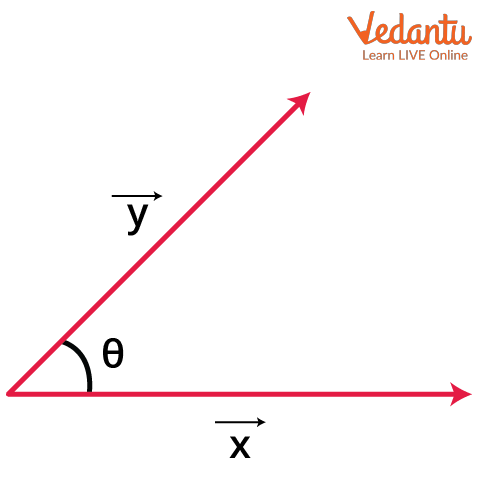Image: Two Vectors x and y

where $\vec x$ and $\vec y$ are two non-linear vectors and $\theta$ is the angle between the two vectors. To find the scalar product of two vectors, we can directly use the scalar product formula mentioned above.

## Important Observations for Scalar Product of Vectors

• The scalar product of two vectors is zero when they are perpendicular to one another. In this case, we also say that the two vectors are orthogonal to each other.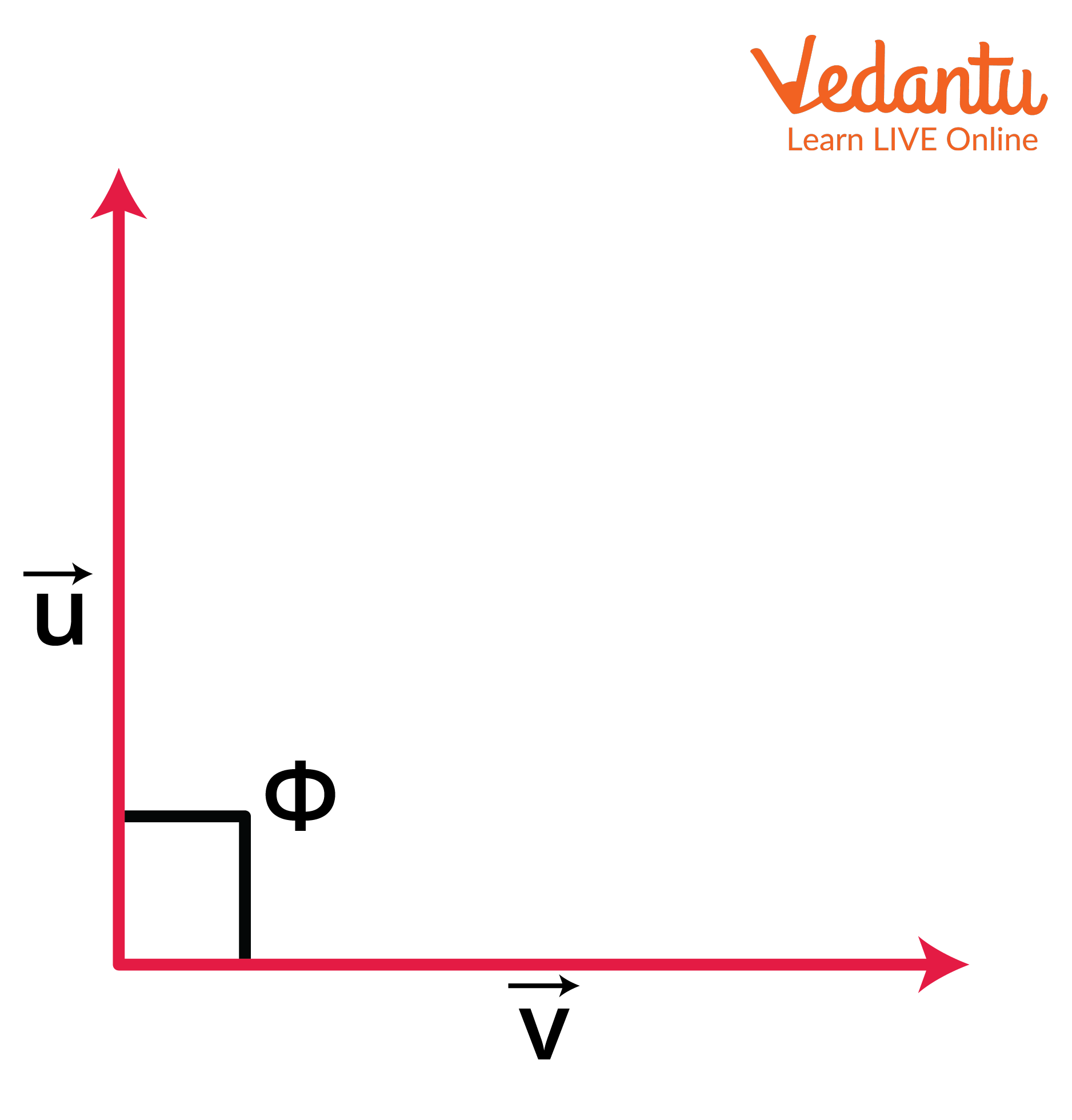Image:  Perpendicular Vectors

• If the two vectors are parallel to each other, then the angle between them is zero. The scalar product of two vectors in this case is simply $\vec{x} \cdot \vec{y}=|\vec{x}| \cdot|\vec{y}|$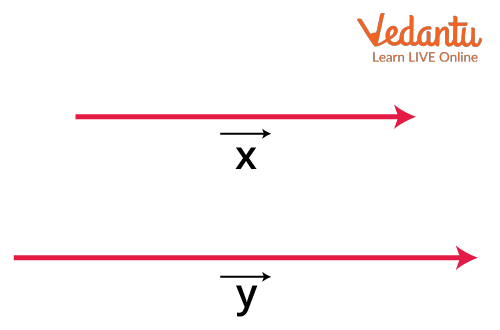Image: Two Parallel Vectors

• If the two vectors are antiparallel to one another when the angle between them is 180o, the scalar product is given as $\vec{x} \cdot \vec{y}=-|\vec{x}||\vec{y}|$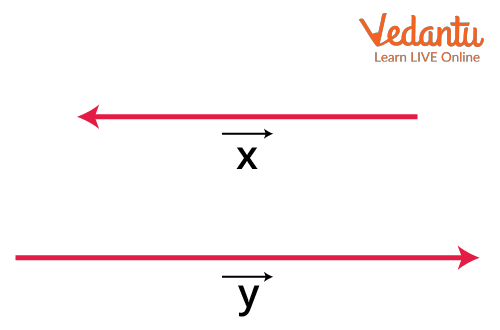Image: Two Anti-Parallel Vectors

## Characteristics of Scalar Product

Some of the important properties of the scalar product of vectors are as listed below:

• Distributive Property

Let $\vec x$, $\vec y$, and $\vec z$ be three vectors. The distributive property of scalar product of the three vectors is denoted as $\vec{x} \cdot(\vec{y}+\vec{z})=\vec{x} \cdot \vec{y}+\vec{y} \cdot \vec{z}$

• Multiplication of Vectors using a Scalar $\lambda$

If $\vec x$, $\vec y$ are two vectors and if the scalar $\lambda$ is multiplied with the dot product of $\vec x$, $\vec y$ we have $\lambda(\vec{x} \cdot \vec{y})=\vec{x} \cdot(\lambda \vec{y}) \text { or }(\vec{x} \lambda) \cdot \vec{y}$

• Commutative Property

If $\vec x$, $\vec y$ are two vectors, then the scalar product of these two vectors is commutative. The commutative property is denoted as $\vec{x} \cdot \vec{y}=\vec{y} \cdot \vec{x}$

### Solved Examples for Scalar Product of Vectors

Example 1: If $\vec x$, $\vec y$ are vectors of two physical quantities with magnitudes 1 and 2, respectively, followed by the scalar product between them being 1, find the angle between the two vectors or physical quantities.

Solution:

Using the formula for scalar product of two vectors, we have

$\cos (\theta)=\dfrac{\vec{x} \cdot \vec{y}}{|\vec{x}||\vec{y}|}$

Given we have $\overline{|\vec{x}||\vec{y}|}=1$ and $\vec{x} \cdot \vec{y}=2$, therefore

\begin{align} &\cos (\theta)=\dfrac{1}{2} \\ &\theta=\cos ^{-1}(0.5) \\ &\theta=60^{\circ} \end{align}

Example 2: If  $\vec{x}=5 \hat{i}+4 \hat{j}+3 \hat{k}$ and $\vec{y}=2 \hat{i}+4 \hat{j}+8 \hat{k}$, show whether the two vectors are perpendicular or not?

Solution:

We know from the above formula that $\cos (\theta)=\dfrac{\vec{x} \cdot \vec{y}}{|\vec{x}||\vec{y}|}$

\begin{align} &\vec{x}=5 \hat{i}+4 \hat{j}+3 \hat{k} \text { and } \vec{y}=2 \hat{i}+4 \hat{j}+8 \hat{k} \\ &\vec{x} \cdot \vec{y}=(5 \hat{i}+4 \hat{j}+3 \widehat{k}) \cdot(2 \hat{i}+4 \hat{j}+8 \widehat{k}) \end{align}

Using the property,

\begin{align} &\vec{x} \cdot \vec{y}=\left(a_{1} i+a_{2} j+a_{3} k\right) \cdot\left(b_{1} i+b_{2} j+b_{3} k\right) \\ &\vec{x} \cdot \vec{y}=a_{1} b_{1}+a_{2} b_{2}+a_{3} b_{3} \\ &\vec{x}=5 \hat{i}+4 \hat{j}+3 \hat{k} \text { and } \vec{y}=2 \hat{i}+4 \hat{j}+8 \hat{k} \\ &\vec{x} \cdot \vec{y}=(5 \hat{i}+4 \hat{j}+3 \hat{k}) \cdot(2 \hat{i}+4 \hat{j}+8 \hat{k}) \\ &\vec{x} \cdot \vec{y}=10+16+24=50 \end{align}

Now, we calculate the magnitude of the two vectors $\vec{x}=5 \hat{i}+4 \hat{j}+3 \hat{k}$ and $\vec{y}=2 \hat{i}+4 \hat{j}+8 \hat{k}$

\begin{align} &\vec{x}=5 \hat{i}+4 \hat{j}+3 \hat{k} \text { and } \vec{y}=2 \hat{i}+4 \hat{j}+8 \hat{k} \\ &|\vec{x}|=\sqrt{5^{2}+4^{2}+3^{2}}=\sqrt{50} \\ &|\vec{y}|=\sqrt{2^{2}+4^{2}+8^{2}}=\sqrt{84} \\ &\text { Thus, }|\vec{x}||\vec{y}|=\sqrt{50} \sqrt{84}=4200 \end{align}

Substituting in the above formula $\cos (\theta)=\dfrac{\vec{x} \cdot \vec{y}}{|\vec{x}||\vec{y}|}$, we get the angle between two vectors as:

\begin{align} &\cos (\theta)=\dfrac{50}{4200} \\ &\cos (\theta)=0.0119 \\ &\theta=\cos ^{-1}(0.0119) \\ &\therefore \theta=89.31^{\circ} \end{align}

Thus, the vectors are not perpendicular to one another.

## Projection Vector

Now that we understand vectors, let us understand how we can project a vector on a line? Suppose a vector XY makes an angle with the line r. If the vector makes an angle in the anticlockwise direction, then the projection of vector XY on this line r will be XY  $\cos{\theta}$. The direction of the vector on the line r will be in the same direction to that of the line, depending on whether the angle is positive or negative. The vector formed on this line is called the projection vector of XY.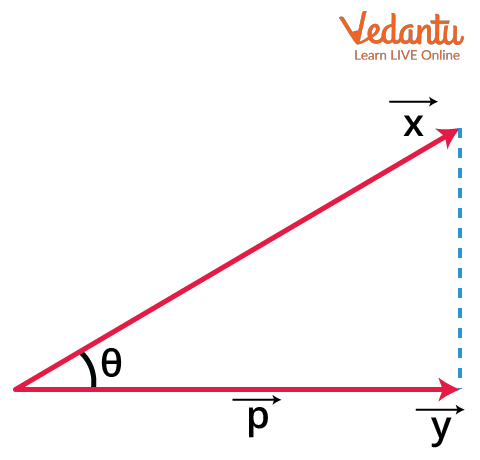Image: Projection of a Vector

## Real-Life Applications of Multiplication of Vectors

Vectors as we know play a fundamental role in Physics, Mathematics, and engineering. We use vectors to define different quantities like force, displacement, momentum, and so on. Now, coming to vector multiplication which is one of the geometric properties of vectors, there are two ways to find the product. One is the cross product and the other one is the dot product. These properties help understand how two or more vectors behave when they are combined together.

Thus, vector multiplication is extensively used in subjects like Physics, mechanics and astronomy, wherein it becomes critical to understand the behaviour of two quantities with both magnitude and direction and how they behave when brought together.

### Conclusion

The scalar product or multiplication of two vectors is usually employed in fields like engineering, mechanics, and geometry. The scalar product of two vectors is also known as the dot product or inner product. The scalar product of two vectors is a scalar and can be solved using the different properties of the scalar product as listed above.

Additional things to keep in mind are the mutually perpendicular unit vectors, i.e., $\hat{i} \cdot \hat{i}=\hat{j} \cdot \hat{j}=\hat{k} \cdot \hat{k}=1$ and $\hat{i} \cdot \hat{j}=\hat{j} \cdot \hat{k}=\hat{k} \cdot \hat{i}=0$. Also, when the angle formed by the vector with the line is 90 degrees, then the projection of the vector will be a zero vector. Thus, the scalar product of vectors is an important concept and must be understood thoroughly.

## FAQs on Scalar Product of Vectors and Its Characteristics for JEE

1. Is pressure a scalar or a vector quantity?

Pressure is denoted as force = pressure × area. Pressure here is nothing but acting as a proportionality factor. Force has a direction, whereas pressure does not. The area of an object or quantity on the other hand decides in which direction the force is to be applied. Thus, pressure has no direction but only magnitude, making it a scalar quantity. We cannot take out components of pressure and find its magnitude. Thus, we can justify that pressure is a scalar quantity.

2. What happens when a vector and a scalar are multiplied?

On multiplying a vector with a scalar, its magnitude changes but the direction keeps changing with the value of the scalar. It means that if the scalar to be multiplied has a value (+) k, the vector direction will be the same whereas if the scalar to be multiplied is (-)k, the vector direction becomes exactly the opposite. For example, we are multiplying a vector $\underset{y}{\rightarrow}$ with a scalar k, then we get  $\underset{K}{\rightarrow}$. On the other hand, when we multiply -k with vector y, the product becomes  $\underset{-K}{\rightarrow}$  . Here, - sign denotes the direction.